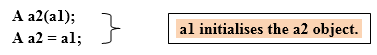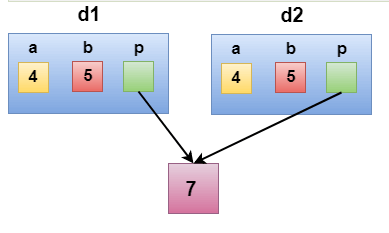# In this tutorial, we are going to learn about the C++  Copy Constructor in OOP, Types of  Copy Constructors in C++, Copy Constructors in C++, C++ Deep Copy, C++ Shallow Copy .

## C++ Copy Constructor in OOP :

A copy constructor is a method or member function which is used to copy data of one object to another. It is usually of the form X (X&), where X is the class name.

Syntax of Copy Constructor in C++ :

## ```Classname(const classname & objectname) { }```

Example of Copy Constructor in C++ :

## ``` class A { A(A &x) // copy constructor.     { // copyconstructor. } };```

In the above case, copy constructor can be called in the following ways:## Uses of Copy Constructor in OOP :

• When we initialize an object by another object of the same class.
• When we return an object as a function value.
• When the object is passed to a function as a non-reference parameter.

## C++ Types of Copy Constructor in OOP:

1. Default Copy Constructor: The compiler defines the default copy constructor. If the user defines no copy constructor, compiler supplies its constructor.

2. User Defined Copy Constructor: The programmer defines the user-defined constructor.

## ``` #include<iostream> using namespace std; class Samplecopyconstructor{ private: int x, y; //data members public: Samplecopyconstructor(int x1, int y1) { x = x1; y = y1; } /* Copy constructor */ Samplecopyconstructor (const Samplecopyconstructor &sam) { x = sam.x; y = sam.y; } void display() { cout<<x<<" "<<y<<endl; }}; /* main function */ int main(){ Samplecopyconstructor obj1(10, 15); // Normal constructor Samplecopyconstructor obj2 = obj1; // Copy constructor cout<<"Normal constructor : "; obj1.display(); cout<<"Copy constructor : "; obj2.display(); return 0; }```

Output :

Normal constructor : 10 15

Copy constructor : 10 15

## When Copy Constructor is called :

Copy Constructor is called in the following scenarios:

When we initialize the object with another existing object of the same class type. For example, Student s1 = s2, where Student is the class.

When the object of the same class type is passed by value as an argument.

When the function returns the object of the same class type by value.

Shallow copy

Deep copy

## C++ Shallow Copy in OOP :

The default copy constructor can only produce the shallow copy.

A Shallow copy is defined as the process of creating the copy of an object by copying data of all the member variables as it is.

Shallow copy constructor is used when class is not dealing with any dynamically allocated memory.

Shallow copy copies references to original objects. The compiler provides a default copy constructor. It is a bit-wise copy of an object.

## ```#include <iostream> using namespace std; class Demo { int a; int b; int *p; public: Demo() { p=new int; } void setdata(int x,int y,int z) { a=x; b=y; *p=z; } void showdata() { std::cout << "value of a is : " <<a<< std::endl; std::cout << "value of b is : " <<b<< std::endl; std::cout << "value of *p is : " <<*p<< std::endl; } }; int main() { Demo d1; d1.setdata(4,5,7); Demo d2 = d1; d2.showdata(); return 0; } ```

Output :

value of a is : 4

value of b is : 5

value of *p is : 7In the above case, a programmer has not defined any constructor, therefore, the statement Demo d2 = d1; calls the default constructor defined by the compiler. The default constructor creates the exact copy or shallow copy of the existing object. Thus, the pointer p of both the objects point to the same memory location. Therefore, when the memory of a field is freed, the memory of another field is also automatically freed as both the fields point to the same memory location. This problem is solved by the user-defined constructor that creates the Deep copy.

## C++ Deep copy in OOP:

Deep copy dynamically allocates the memory for the copy and then copies the actual value, both the source and copy have distinct memory locations. In this way, both the source and copy are distinct and will not share the same memory location. Deep copy requires us to write the user-defined constructor.

Deep copy allocates separate memory for copied information.

## General requirements for deep copy:

· A normal constructor.

· A destructor to delete the dynamically allocated memory.

· A copy constructor to make a copy of the dynamically allocated memory.

## ```#include <iostream> using namespace std; class Demo { public: int a; int b; int *p; Demo() { p=new int; } Demo(Demo &d) { a = d.a; b = d.b; p = new int; *p = *(d.p); } void setdata(int x,int y,int z) { a=x; b=y; *p=z; } ~Demo() { delete p; } void showdata() { std::cout << "value of a is : " <<a<< std::endl; std::cout << "value of b is : " <<b<< std::endl; std::cout << "value of *p is : " <<*p<< std::endl; } }; int main() { Demo d1; d1.setdata(4,5,7); Demo d2 = d1; d2.showdata(); return 0; } ```

Output :

value of a is : 4

value of b is : 5

value of *p is : 7

In the above case, a programmer has defined its own constructor, therefore the statement Demo d2 = d1; calls the copy constructor defined by the user. It creates the exact copy of the value types data and the object pointed by the pointer p. Deep copy does not create the copy of a reference type variable.